# C++ - Boolean Logics with STL

### Logic gates or circuits are devices that operate and work with one or more logic input signals to produce one and only one output.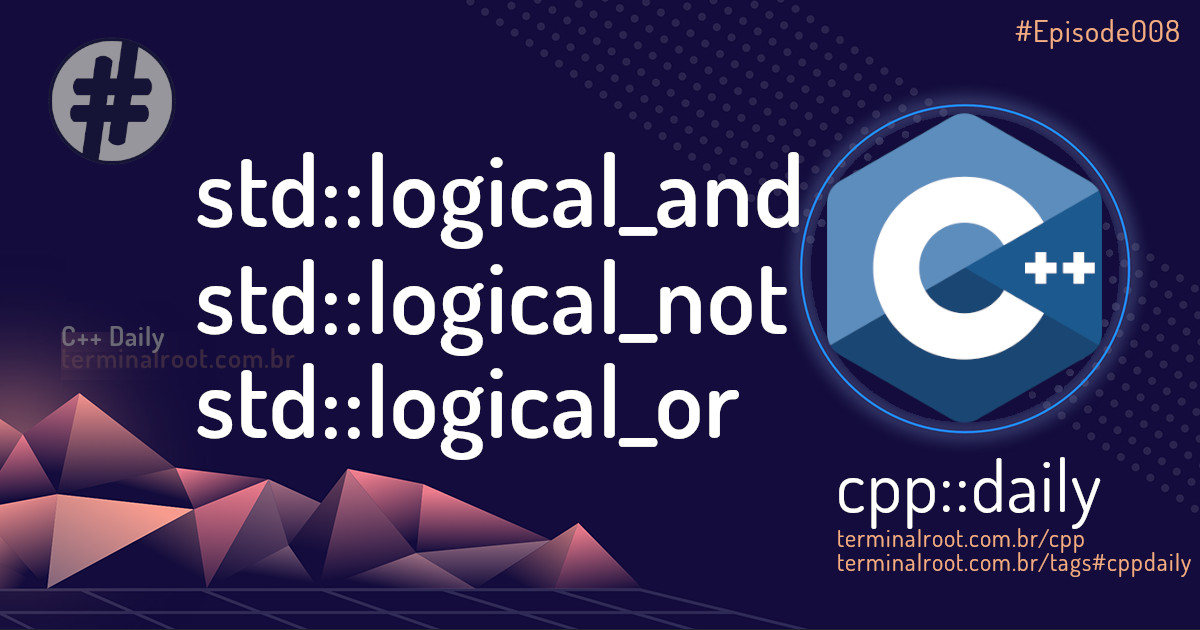Logic gates or circuits are devices that operate and work with one or more logic input signals to produce one and only one output, depending on the function implemented in the circuit.

The situations “Truth” and “False” are studied in the Mathematical Logic or Boole Logic. There are the following logic gates: AND, OR and NOT are the main ones, because with them we can form the others that are: NAND, NOR, XOR and XNOR.

Remembering that when analyzing execution output: 0 is true (without error) and 1 is false ( >= 1 is error ), so this can confuse your mind when analyzing the examples, as it is the other way around, but I will not invert because it is as soon as it is in reality, then we will use the concept of computation and not execution output: 0 as false and 1 is true. 😃

# AND `logical_and`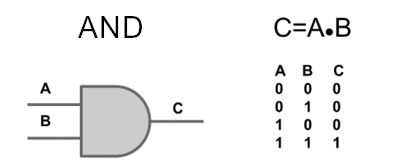This port results in a true logical value only if all operators have a true value (in this case it is 1). An idea of understanding would be two switches connected in series) and a lamp at the end, that is, the lamp will only be switched on if these two switches are on.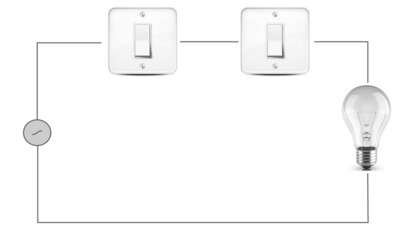For these examples we will use the `std::transform` function. Let’s analyze the output of the combination of two arrays using `std::logical_and`:

The output will be:

If you want to see the literal output, use this:

The output will be:

That is, just like the example of the lamps, only if both are true that the output is true!

# NOT `logical_not`

The NOT or inverter port is a digital logic port that implements logical negation, according to the truth table below. That is, if it is false it returns true and if it is true it returns false.

In this example we will use only one array (only with 2 elements) and of course we will only pass 4 parameters to `std::transform`.

The output will be:

# OR `logical_or`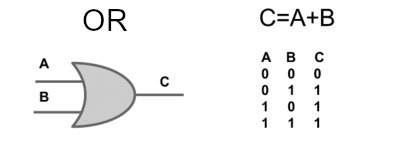The OR logic gate is also called a logical disjunction, it is a logical operation between two or more operands that results in a false logical value if, and only if, all operands have a false value.

That is, if all values are false it will be false, otherwise it will be true.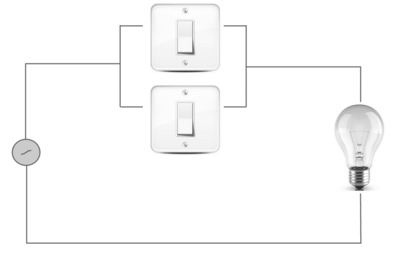Returning to the example of analyzing 2 arrays.

The output will be:

That’s it for today, they are small daily doses that will always keep us tuned in to C++!